# KSEEB Solutions for Class 9 Science Chapter 11 Work and Energy

In this chapter, we provide KSEEB SSLC Class 9 Science Chapter 11 Work and Energy for English medium students, Which will very helpful for every student in their exams. Students can download the latest KSEEB SSLC Class 9 Science Chapter 11 Work and Energy pdf, free KSEEB SSLC Class 9 Science Chapter 11 Work and Energy pdf download. Now you will get step by step solution to each question.

### KSEEB Solutions Class 9 Science Chapter 11 Intext Questions

Question 1.
A force of 7N acts on an object. The displacement is, say 8m, in the direction of the force. Let us take it that they force acts on the object through the displacement. What is the work done in this case?
Work done on an object = 7N × 8m = 56 Nm or 56 J.

Question 2.
When do we say that work is done?
Two conditions need to be satisfied for work to be done:

1. a force should act on an object and
2. the object must be displaced.

Question 3.
Write an expression for the work done when a force is acting on an object in the direction of its displacement.
Let a constant force F act on an object. Let the object be displaced through a distance S in the direction of the force. Let W be work done. We define work to be equal to the product of the force and displacement.
Work done = force × displacement W = F × S.

Question 4.
Define 1 J of work.
1 J is the amount of work done on an object when a force of 1 N displaces it by 1 m along the line of action of the force.

Question 5.
A pair of bullocks exerts a force of 140 N on a plough. The field being ploughed is 15m long. How much work is done in ploughing the length of the field?
Force exerted, F = 140N.
Displacement, S = 15m
Work done in ploughing the field
W = F × S
= 140 × 15
= 2100J
= 2.1 × 103 J.

Question 6.
What is the kinetic energy of an object?
Objects in motion possess energy, we call this energy kinetic energy.

Question 7.
Write an expression for the kinetic energy of an object.
KE = 12 mv2.

Question 8.
The kinetic energy of an object of mass, m moving with a velocity of 5 ms-1 is 25 J. What will be its kinetic energy when its velocity is doubled? What will be its kinetic energy when its velocity is increased three times?
Kinetic energy, k = 12 mv2.
Where m = mass of the object
V = velocity of the object.
Here mass (m) is the same in both cases (∵ object is same)
k1k2=(v1v2)2
Initial kinetic energy k1 = 25J
Initial velocity V2 = 5 ms-1
New kinetic Energy K2 = ?
New velocity v2 = 3v1 = 3 × 5 = 10 ms-1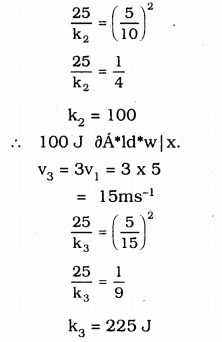∴ When velocity is increased three times its K.E. is 225J.

Question 9.
What is power?
Power is defined as the rate of doing work or the rate of transfer of energy w
P = wt

Question 10.
Define 1 watt of power.
Power is 1 W when the rate of consumption of energy is 1 JS-1.

Question 11.
A lamp consumes 1000 J of electrical energy in 10s. What is its power?
Power = 1000 J Work w = 1000J
Time = 10 s
Power of lamp, P = w/t
= 1000/10 = 100W
∴ Power of a lamp = 100 KW.

Question 12.
Define average power.
We obtain average power by dividing the total energy consumed by the total time taken.

### KSEEB Solutions for Class 9 Science Chapter 11 Textbook Exercises

Question 1.
Look at the activities listed below. Reason out whether or not work is done in the light of your understanding of the term ‘work’

1. Suma is swimming in a pond.
2. A donkey is carrying a load on its back.
3. A windmill is lifting water from a well.
4. A green plant is carrying out photosynthesis.
5. An engine is pulling a train.
6. Food grains are getting dried in the sun.
7. A sailboat is moving due to wind energy.

1. Work is being done by Seema because she displaces the water by applying the force.
2. No work is being done by the donkey because the direction of force i.e the load is vertically downward and displacement is along the horizontal. If displacement and force are perpendiculars then no work is done.
3. Work is done because the windmill is lifting the water i.e., it is changing the position of water.
4. No work is done because there are no force and displacement.
5. Work is done because the engine is changing the position of the train.
6. No work’ is done because there is no force and no displacement.
7. Work is done because the force acting on the boat is moving it.

Question 2.
An object was thrown at a certain angle to the ground moves in a curved path and falls back to the ground the initial and the final points of the path of the object lie on the same horizontal line. What is the work done by the force of gravity on the object?
Work done by the force of gravity, W = mgh.
Where h = difference in height of initial and final positions of the object.
According to the question, the initial and final positions of the object lie in the same horizontal line. So h = 0.
∴ Work done W = mg × 0 = 0

Question 3.
A battery lights a bulb. Describe the energy changes involved in the process.
In the case given in the question, the battery has chemical energy which is converted into energy. Electric energy provided to the bulb further converted into light energy.

Question 4.
A certain force acting on a 20 kg mass changes its velocity from 5 ms-1 to 2 ms-1. Calculate the work done by the force.
Mass, m = 20 kg.
Initial velocity, u = 5 ms-1
Final velocity v = 2ms-1
Work done by the force = change in kinetic energy
= Final kinetic energy – Initial kinetic energy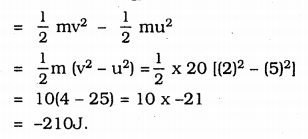Question 5.
A mass of 10kg is at a point A on a table. It is moved to a point B. If the line joining A and B is horizontal what is the work done on the object by the gravitational force? Explain your answer.
Work done by gravitational force W = mgh
Where h = Difference in the heights of initial and final positions of the object.
Here both the initial and final positions are on the same horizontal line.
So there is no difference in height i.e., h = 0.
∴ work done W = mg × 0 = 0.

Question 6.
The potential energy of a freely falling object decreases progressively. Does this violate the law of conservation of energy? Why?
The total mechanical energy remains constant / as P.E. of the freely falling object decreases. Its kinetic energy and the kinetic energy increases on account of an increases its velocity) the law of conservation of energy is not violated.

Question 7.
What are the various energy transformations that occur when you are riding a bicycle?

1. Muscular energy into kinetic energy
2. the kinetic energy of the rotation of the which into kinetic energy of the bicycle.

Question 8.
Does the transfer of energy take place when you push a huge rock with all your might and fail to move it? Where is the energy you spend going?
When we push a huge rock and fall to move it. The energy spent in doing so is absorbed by the.rock. This energy is converted into potential energy of the configuration of the rock which results in its deformation. However t his deformation is not visible on account of the huge size of the rock.

Question 9.
A certain household has consumed 250 units of energy during a month. How much energy is this in Joules?
Energy consumed w = 250 units
= 250 kwh
= 250 × 1000 W × 3600 S
= 250 × 1000 J/S × 3600 S
= 9 × 108 J.

Question 10.
An object of mass 40 kg is raised to a height of 5m above the ground what is its potential energy? If the object is allowed to fall, find its kinetic energy when it is halfway down.
Mass m = 40 kg.
height h = 5 m.
Potential energy PE = mgh = 40 × 9.8 × 5 = 1960J.
KE at half way down = PE at halfway down = mgh2
= 40 × 9.8 × 52 = 980 J.

Question 11.
What is the work done by the force of gravity on a satellite moving round the earth? Justify your answer.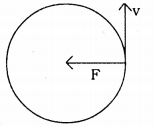The satellite round the earth moves in a circular orbit. Here the force of gravity acts towards the centre of the earth and displacement of the satellite is along the tangent of the circular path that means therefore and displacement are perpendicular to each other.
So, work done, W = F.S cosθ
= F × S cos 90°
= F × S × 0
= 0
That is, no work is done by the force of gravity.

Question 12.
Can there be displacement of an object in the absence of any force acting on it? think. Discuss this question with your friends and teacher.
If an object moves with a constant velocity (i.e, there is no acceleration) then no force acts on it. As the object is moving ie it is displaced from one position to another position.

Question 13.
A person holds a bundle of hay over his head for 30 minutes and gets tired. Has he done some work or not? Justify your answer.
The person has no movement ie his displacement is zero. So the person had done no work (∵ work is done only when the object is displaced).

Question 14.
An electric heater is rated 1500 W. How much energy does it use in 10 hours?
Time = 10 h = 10 × 60 min
= 10 × 60 × 60 S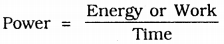Energy = power × time
= 1500 × 10 × 60 × 60
= 5.4 × 107 J.

Question 15.
Illustrate the law of conservation of energy by discussing the energy changes which occur when we draw a pendulum bob to one side and allow it to oscillate why does the bob eventually comes to rest? What happens to its energy eventually? Is it a violation of the law of conservation of energy?
When the pendulum oscillates in the air, the air friction opposes its motion. So some part of the kinetic energy of the pendulum is used to overcome this friction. With the passage of time, the kinetic energy of the pendulum goes on decreasing and finally becomes zero. The kinetic energy of the pendulum is transferred to the atmosphere. So energy is being transferred ie it is converted into one form to another. So here is no violation of the law of conservation of energy.

Question 16.
An object of mass, m is moving with a constant velocity, v. How much work should be done on the object in order to bring the object to rest?
The work done on the object to bring the object to rest
= change in kinetic energy
= Final kinetic energy – Initial Kinetic energy
Here final kinetic energy is zero because the object is brought to rest.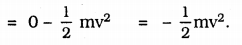Question 17.
Calculate the work required to be done to stop a car of 1500 kg moving at the velocity of 60km/h?
Mass, m = 1500 kg.
Initial velocity, u = 60 kmh-1
= 60 × 518 = 16.67 ms-1
Final velocity, v = 0
(∵ the car comes to rest)
Work done to stop the car = change in kinetic energy
= Final kinetic energy – Initial Kinetic energy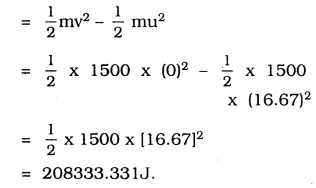Question 18.
In each of the following a force, F is acting on an object of mass M. The direction of displacement is from west to east shown by the longer arrow. Observe the diagram carefully and state whether the work done by the force is negative, positive or zero.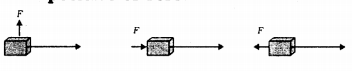Cas i) The force and displacement are perpendicular to each other. So S = 90°
Work done = FScos θ°
= FScos 90°
= FS × 0 = 0
(∵ cos90° = 0)
Cas ii) The force and displacement are in the same direction so θ = 0°
Work done = FScos θ°
= FScos 90°
= FS × 1 = FS
(∵ cos θ° = 1)
That is the work done is positive.
Cas iii) The force and displacement in opposite direction θ = 180°
Workdone = FScos θ°
= FScos 180°
= FS × -1 = FS
(∵ cos 180° = -1)
That is workdone is negative.

Question 19.
Soni says that the acceleration in an object could be zero even when several forces are acting on it. Do you agree with her? Why?
Yes, I agree with Soni, the acceleration of an object can be zero even when several forces are acting on it if the resultant of all the forces acting is zero.

Question 20.
Find the energy in kWh consumed in 10 hours by four devices of power 500 w each.
Total power p = 500w × 4 = 2000w.
Time, t = 10h
Energy = p × t.
= 2000w × 10h
= 2kwh × 10h = 20kwh.
∴ Energy = 20kwh.

Question 21.
A freely falling object eventually stops on reaching the ground. What happens to its kinetic energy?
P.E. of the configuration of the body and the ground (the body may be deformed and the ground may at the place of collision).
This process energy in which the kinetic energy of a freely falling body is lost in an unproductive chain of energy charges is called dissipation of energy.

### KSEEB Solutions for Class 9 Science Chapter 11 Additional Questions and Answer

Fill in the blanks:
Question 1.
Work done = ___________
force, displacement

Question 2.
An object having the capability to do work is said to possess __________
energy.

Question 3.
__________ is the energy possessed by an object due to its motion.
Kinetic energy

Question 4.
P.E
mgh.

Question 5.
Larger unit of energy is called
kilojoule.

Question 1.
Can there be displacement of an object in the absence of any force acting on it?
In the absence of any force on the object i.e., F = 0, ma, (as F = ma) since m ≠ 0 and a = 0. In such a case the object is either at rest or in a state of uniform motion in a straight line in the latter case there is a displacement . of the object without any force acting on it.

Question 2.
How does a bullet pierce a target?
A bullet moves with large velocity and as such possesses a lot of kinetic energy the work in piercing the target is derived from the kinetic energy of the bullet.

Question 3.
Why do some engines require fuels like petrol and diesel?
Internal combustion heat engines use the chemical energy of fossil fuel (petrol and diesel) for their operation. These engines first convert the chemical energy of the fuels into heat energy. Which is later on converted into mechanical energy.

Question 4.
Calculate the work done by a body. By the force of 5 N makes to move through a distance of 12 m.
W = F x s = 5 x 12 = 60 joules

Question 5.
When force 6 N applied on a wall, the wall remains in the same position, calculate the work done.
W = F x s
W – 6 x s = 6 x 0 = 0 Joules No work is done on the body

Question 6.
Electricity is the most convenient form of energy. Why?
It can be converted into other forms of energy easily.
It can be produced by different means. It is ecofriendly

Question 7.
Calculate the power experienced by a source that can do work of 50 joules in 5 seconds.
Power = \$ Workdone  Time taken \$
=505 Joules  Second  = 10 J/s = 10 watts

All Chapter KSEEB Solutions For Class 9 Science

—————————————————————————–

All Subject KSEEB Solutions For Class 9

*************************************************

I think you got complete solutions for this chapter. If You have any queries regarding this chapter, please comment on the below section our subject teacher will answer you. We tried our best to give complete solutions so you got good marks in your exam.

If these solutions have helped you, you can also share kseebsolutionsfor.com to your friends.

Best of Luck!!